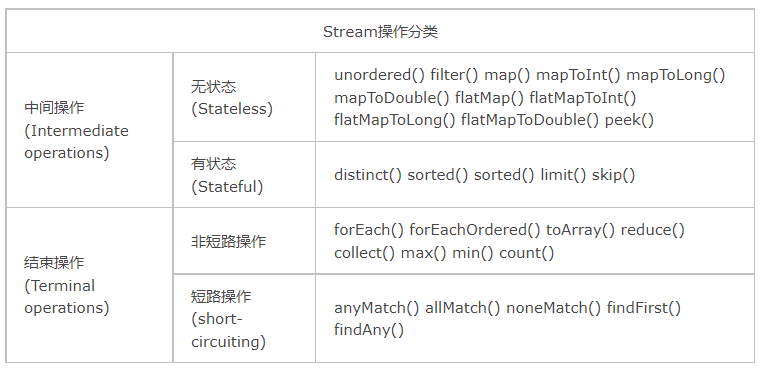# #夏日挑战赛# Java 8 Stream 从入门到原理 原创精华

1收藏

## 0.阅读完本文你将会

• 了解Stream的定义和它的特征
• 了解Stream的基础和高阶用法

## 2. 流的定义

A Stream is a sequence of elements from a source.

``````+--------------------+       +------+   +------+   +---+   +-------+
| stream of elements +-----> |filter+-> |sorted+-> |map+-> |collect|
+--------------------+       +------+   +------+   +---+   +-------+
``````1. 流并不存储数据，所以它不是一个数据结构，它也不会修改底层的数据源，它为了函数式编程而生。

2. 惰性执行的，例如filter,map等都是延迟执行的。流的中间操作总是惰性的。

``````List<String> list = Arrays.asList("a", "b", "c");
Stream<String> stream = list.stream().filter(element -> {
System.out.println("filter() was invoked");
return element.contains("b");
});
``````

``````List<String> list = Arrays.asList("a", "b", "c");
Optional<String> stream = list.stream().filter(element -> {
System.out.println("filter() was invoked");
return element.contains("b");
}).map(ele -> {
System.out.println("map() was invoked");
return ele.toUpperCase();
}).findFirst();
``````

``````filter() was invoked
filter() was invoked
map() was invoked
``````

1. 流有可能是无限的。虽然集合具有有限的大小，但流不需要。短路操作，如limit(n)或findFirst()，允许在有限的时间内完成对无限流的计算。

2. 流还是消耗品。在流的生命周期中，流的元素只被访问一次。与迭代器一样，必须生成新的流来重新访问源的相同元素。被访问过的流会被关闭。

``````IntStream intStream = IntStream.of(1, 2, 3);
OptionalInt anyElement = intStream.findAny();
OptionalInt firstElement = intStream.findFirst();
``````

``````Exception in thread "main" java.lang.IllegalStateException: stream has already been operated upon or closed
``````

``````int[] intArray = {1, 2, 3};
OptionalInt anyElement = Arrays.stream(intArray).findAny();
OptionalInt firstElement = Arrays.stream(intArray).findFirst();
``````

## 3. 创建一个流

### 3.1 创建一个空的流

``````Stream<String> emptyStream = Stream.empty();
``````

``````public Stream<String> streamOf(List<String> list) {
return list == null || list.isEmpty() ? Stream.empty() : list.stream();
}
``````

### 3.2 使用数组创建流

``````String[] arr = new String[]{"1", "2", "3"，"4", "5"};
Stream<String> entireArrayStream = Arrays.stream(arr);
Stream<String> partArrayStream = Arrays.stream(arr, 1, 4);
``````

### 3.3 使用集合创建流

``````Collection<String> collection = Arrays.asList("1", "2", "3");
Stream<String> collectionStream = collection.stream();
``````

### 3.4 使用Stream.Builder()来创建流

``````Stream<String> streamBuilder =
``````

### 3.5 使用File来创建流

``````Path path = Paths.get("C:\\tmp\\file.txt");
Stream<String> fileStream = Files.lines(path);
Stream<String> fileStreamWithCharset = Files.lines(path, Charset.forName("UTF-8"));
``````

### 3.6 Stream.iterate()

``````Stream<Integer> iteratedStream = Stream.iterate(10, n -> n + 1).limit(10);
``````

### 3.7 Stream.generate()

generate() 方法接受一个`Supplier<T>`来生成元素。

``````Stream<String> generatedStream =
Stream.generate(() -> "ele").limit(5);
``````

### 3.8 基本类型的流

1. range()和rangeClosed()

``````IntStream intStream = IntStream.range(1, 3);//1,2
LongStream longStream = LongStream.rangeClosed(1, 3);//1,2,3
``````

range(int start, int end) 方法会创建一个从start到end的有序流，它的步长是1，但是它不包括end。

rangeClosed(int start, int end)range() 方法的区别在于，前者会包括end。

2. of()方法

``````int[] intArray = {1,2,3};
IntStream intStream = IntStream.of(intArray);//1,2,3
IntStream intStream2 = IntStream.of(1, 2, 3);//1,2,3

long[] longArray = {1L, 2L, 3L};
LongStream longStream = LongStream.of(longArray);//1,2,3
LongStream longStream2 = LongStream.of(1L, 2L, 3L);//1,2,3

double[] doubleArray = {1.0, 2.0, 3.0};
DoubleStream doubleStream = DoubleStream.of(doubleArray);
DoubleStream doubleStream2 = DoubleStream.of(1.0, 2.0, 3.0);//1.0,2.0,3.0
``````

3. Random类

``````Random random = new Random();
IntStream intStream = random.ints(3);
LongStream longStream = random.longs(3);
DoubleStream doubleStream = random.doubles(3);
``````

### 3.9 字符串的流

1. 字符的流

``````IntStream charStream = "abc".chars();
``````

2. 字符串的流

``````Stream<String> stringStream = Pattern.compile(",").splitAsStream("a,b,c");
``````

## 4. 流的用法

### 4.1 基本用法

#### 4.1.1 forEach()方法

``````Arrays.asList("Try", "It", "Now")
.stream()
.forEach(System.out::println);
``````

``````Try
It
Now
``````

1. 方法引用

``````Arrays.asList("Try", "It", "Now")
.stream()
.forEach(ele -> System.out.println(ele));
``````

`System.out::println`就是引用了某个对象的方法。

2. 副作用

``````// 错误
List<String> list = new ArrayList<>();
stream.filter(s -> pattern.matcher(s).matches())
// 正确
List<String> list2 =
stream.filter(s -> pattern.matcher(s).matches())
.collect(Collectors.toList());//无副作用

``````

#### 4.1.2 filter()方法

filter() 方法的作用是返回符合条件的Stream。

``````Arrays.asList("Try", "It", "Now")
.stream()
.filter(ele -> ele.length() == 3)
.forEach(System.out::println);
``````

``````Try
Now
``````

#### 4.1.3 distinct()方法

distinct() 方法返回一个去重的stream。

``````Arrays.asList("Try", "It", "Now", "Now")
.stream()
.distinct()
.forEach(System.out::println);
``````

#### 4.1.4 sorted()方法

``````Arrays.asList("Try", "It", "Now")
.stream()
.sorted((str1, str2) -> str1.length() - str2.length())
.forEach(System.out::println);
``````

``````It
Try
Now
``````

#### 4.1.5 map()方法

map() 方法对每个元素按照某种操作进行转换，转换后流的元素不会改变，但是元素类型取决于转换之后的类型。

``````Arrays.asList("Try", "It", "Now")
.stream()
.map(String::toUpperCase)
.forEach(System.out::println);
``````

``````TRY
IT
NOW
``````

#### 4.1.6 flatMap()方法

flat的英文就是”平坦的“意思，而flatMap()方法的作用就是将流的元素摊平，借助下面这个例子我们更好理解：

``````Stream.of(Arrays.asList("Try", "It"), Arrays.asList("Now"))
.flatMap(list -> list.stream())
.forEach(System.out::println);
``````

``````Try
It
Now
``````

### 4.2 归约操作

Stream类库有两个通用的归约操作reduce()和collect() ，也有一些为简化书写而设计的专用归约操作，比如sum()、max()、min()、count()等。

#### 4.2.1 reduce()

reduce操作可以实现从一组元素中生成一个值，比如sum()、max()、min()、count()等都是reduce操作。

reduce()方法定义有三种形式：

``````Optional<T> reduce(BinaryOperator<T> accumulator)

T reduce(T identity, BinaryOperator<T> accumulator)

<U> U reduce(U identity, BiFunction<U,? super T,U> accumulator, BinaryOperator<U> combiner)
``````

1. identity-初始值

2. accumulator-累加器

3. combiner-拼接器，只有并行执行时才会用到。

``````Optional<Integer> reducedInt = Stream.of(1, 2, 3).reduce((a, b) -> a + b);
``````

reducedInt = 1 + 2 + 3 = 6

``````int reduceIntWithTwoParams = Stream.of(1, 2, 3).reduce(10, (a, b) -> a + b);
``````

reduceIntWithTwoParams = 10 + 1 + 2 + 3 = 16

``````int reducedIntWithAllParams = Stream.of(1, 2, 3).reduce(10, (a, b) -> a + b, (a, b) -> {
System.out.println("Combiner was invoked.");
return a + b;
});
``````

``````int reducedIntWithAllParams = Arrays.asList(1, 2, 3).parallelStream().reduce(10, (a, b) -> a + b, (a, b) -> {
System.out.println("Combiner was invoked");
return a + b;
});
``````

reducedIntWithAllParams = (10 + 1)+ ((10 + 2) + (10 + 3)) = 36

Collection.stream() 和 Collection.parallelStream() 分别产生序列化流(普通流)和并行流。

#### 4.2.2 collect()方法

collect()应该算是Stream里的最终王牌选手了，基本上你想要的功能都能在这里找到。

``````List<Student> students = Arrays.asList(new Student("Jack", 90)
, new Student("Tom", 85)
, new Student("Mike", 80));
``````

1. 常规归约操作

• 获取平均值
``````Double averagingScore = students.stream().collect(Collectors.averagingDouble(Student::getScore));
``````
• 获取和
``````Double summingScore = students.stream().collect(Collectors.summingDouble(Student::getScore));
``````
• 获取分析数据
``````DoubleSummaryStatistics doubleSummaryStatistics = students.stream().collect(Collectors.summarizingDouble(Student::getScore));
``````

Collectors提供的这些方法省去了额外的map() 方法，当然你也可以先使用map() 方法，再进行操作。

2. 将流转换成Collection

``````List<String> studentNameList = students.stream().map(Student::getName).collect(Collectors.toList());//[Jack, Tom, Mike]
``````

``````String studentNameList = students.stream().map(Student::getName).collect(Collectors.joining(",", "[", "]"));//打印出来就是[Jack,Tom,Mike]
``````

3. 将流转换成Map

Map不能直接转换成Stream，但是Stream生成Map是可行的，在生成Map之前，我们应该先定义好Map的Key和Value分别代表什么。

• Collectors.toMap(),使用者需要指定Map的key和value；
• Collectors.groupingBy(),对元素进行group操作；
• Collectors.partitioningBy()，对元素进行二分区操作。

Collectors.toMap()

``````Map<Student, Double> collect = students
.stream()
.collect(Collectors.toMap(Function.identity(), Student::getScore));
``````

Collectors.groupingBy()

``````Map<Double, List<Student>> nameStudentMap = students.stream().collect(Collectors.groupingBy(Student::getScore));
``````

Collectors.partitioningBy()

partitioningBy()按照某个二元逻辑将stream中的元素分为两个部分，比如说下面这个例子将Student分成了成绩及格或者不及格的部分。

``````Map<Boolean, List<Student>> map = students.stream().collect(Collectors.partitioningBy(ele -> ele.getScore() >= 85));
``````

``````{false=[Student{name='Mike', score=80.0}], true=[Student{name='Jack', score=90.0}, Student{name='Tom', score=85.0}]}
``````

## 5. 结语

Java 8 Stream是一个强大的工具，但是我们在使用它的时候一定要符合规范，不然它可能会给你带来意想不到的惊喜哦~

6

8条回复

/

longlong899

2022-7-8 09:56:47

2022-7-30 15:02:33

2022-7-30 15:04:34

2022-7-30 15:08:47

2022-7-30 15:11:00

2022-7-30 15:12:35帖子
视频
声望
粉丝
热门推荐
社区精华内容